# Nonlinear Equations (4 Types You Should Know, Plus How To Solve)

Nonlinear equations appear often in algebra, calculus, and physics (for example, when solving problems that involve gravity or acceleration).  Since they are pretty common, it helps to know about nonlinear equations and how to solve them.

So, what are nonlinear equations?  A nonlinear equation has at least one term that is not linear or constant. It cannot be reduced to the forms ax + b = 0 or y = ax + b. Nonlinear equations can contain polynomials with quadratic, cubic, and higher order terms. They can also contain rational, exponential, and logarithmic functions.

Of course, you can take two or more nonlinear systems together in order to get a nonlinear system.  You can sometimes solve these systems to find one or more solutions (although some have no solution).

In this article, we’ll take a look at nonlinear equations, what they are, and how to solve them.  We’ll also look at nonlinear systems, along with examples to make the concepts clear.

Let’s get started.

## What Are Nonlinear Equations?

A nonlinear equation has at least one term that is not linear or constant.  Remember that:

• A constant term has no variables – for example, 2, 7, 4.5, etc.
• A linear term has a variable raised to the first power – for example, x, 5.4y, 8z, etc.

Since a linear equation has the form ax + b = 0 (or y = ax + b), we know that a nonlinear equation does not have this form.

There are several types of nonlinear equations, each of which has a different solution method.

### Types Of Nonlinear Equations

Here are some types of nonlinear equations that you may see:

• Polynomial of degree N > 1: these equations have quadratic, cubic, quartic, quantic, or higher order terms (involving powers of x2, x3, x4, x5, etc.)
• Radical or Rational Exponent: these equations have terms with variables that have exponents that are not whole numbers (such as the terms x1/2 = √x, x0.3, x7/3, etc.)
• Rational Function: these equations have fractions with polynomials in the numerator and denominator (such as 4 / x, (x – 3) / (x – 2), etc.). You can learn more about the domain and range of a rational function here.
• Exponential: these equations have terms with a constant base and a variable in the exponent (such as 2x, ex, 3x + 2, etc.)
• Logarithmic: logarithms are just another way of talking about exponents.  These equations have terms with a logarithm (such as log2(x), log10(x + 2), etc.)
• Trignonometric: these equations include terms with sine, cosine, and tangent (such as sin(x), cos(2x), etc.)
• Combination: these equations have some combination of the types of terms above (such as (4 / x) = 2x + 5.  These can be very difficult to solve without a computer.

### How To Solve A Nonlinear Equation

Let’s take a look at some examples of nonlinear equations and how to solve them.

#### Example 1: Solving A Nonlinear Equation

Let’s say we want to solve the following nonlinear equation:

• x3 + 5x2 + 6x = 0

This is a nonlinear equation that includes a cubic polynomial (a cubic equation).  The first thing to notice is that we can factor out an x (don’t divide by x, since we could lose the possible solution x = 0):

• x(x2 + 5x + 6) = 0

We can factor the quadratic expression in parentheses as follows:

• x(x + 2)(x + 3) = 0

So, the three solutions of this cubic equation are x = 0, x = -2, and x = -3. We can verify this in the graph below: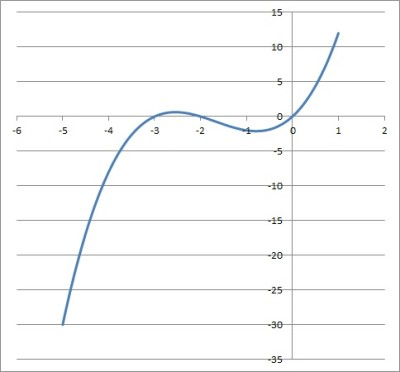The graph of the nonlinear equation x3 + 5x2 + 6x = 0 has solutions at x = 0, x = -2, and x = -3.

#### Example 2: Solving A Nonlinear Equation

Let’s say we want to solve the following nonlinear equation:

• √x + 2 = x

This is a nonlinear equation that includes a radical (a radical equation).  The first thing to notice is that we can isolate the radical term on the left side by subtracting 2 from both sides:

• √x = x – 2

Now we can square both sides to cancel the radical (since √x = x1/2) :

• (√x)2 = (x – 2)2

The equation simplifies to:

• x = x2 – 4x + 4
• 0 = x2 – 5x + 4
• 0 = (x – 1)(x – 4)

So, the two solutions of this radical equation are x = 1 and x = 4.

We can check both in the original equation to be sure.

For x = 1:

• √x = x – 2
• √1 = 1 – 2
• 1 = -1

This is not true, so x = 1 is an extraneous solution. (You can learn more about extraneous solutions and how they emerge here).

For x = 4:

• √4 = 4 – 2
• 2 = 2

This is true, so x = 4 is the only solution. We can verify this in the graph below: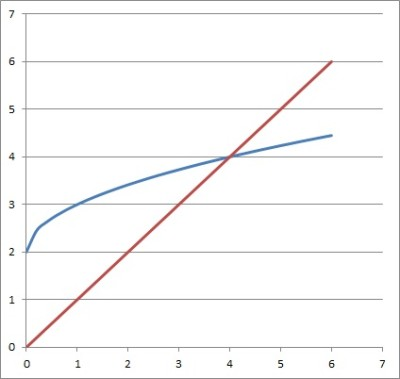The functions y = √x + 2 (blue curve) and y = x (red line) intersect at x = 4.

#### Example 3: Solving A Nonlinear Equation

Let’s say we want to solve the following nonlinear equation:

• (4 / x) – x = 3

This is a nonlinear equation that includes a rational term (a rational equation).  The first thing to notice is that we can clear the denominator if we multiply by x on both sides:

• (4 / x)*x – x*x = 3x

After simplifying, we get:

• 4 – x2 = 3x

Rearranging terms, we get:

• 0 = x2 + 3x – 4

Factoring the right side gives us:

• 0 = (x – 1)(x + 4)

So, the two solutions of this rational equation are x = 1 and x = -4.

We can also check these answers in the original equation (both of them are valid). We can verify this in the graph below: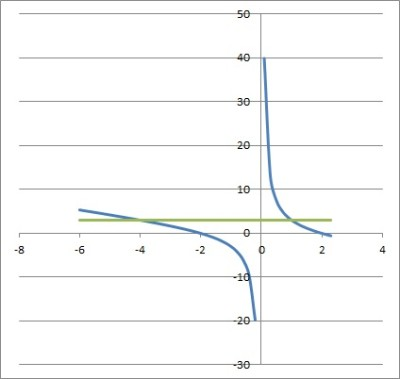The functions y = (4 / x) – x (blue curve) and y = 3 (green line) intersect at x = -4 and x = 1.

#### Example 4: Solving A Nonlinear Equation

Let’s say we want to solve the following nonlinear equation:

• 4x – 2x+3 + 7 = 0

This is a nonlinear equation that includes exponential terms (an exponential equation).  The first thing to notice is that we can change the base for the first term, 4, to match the base of the second term, 2:

• (22)x – 2x+3 + 7 = 0
• 22x – 2x+3 + 7 = 0

Now we can write the first two terms in terms of 2x:

• (2x)2 – 23*2x + 7 = 0
• (2x)2 – 8*2x + 7 = 0

Now, we can substitute t = 2x to give us a more familiar equation:

• t2 – 8t + 7 = 0

Now we factor the left side:

• (t – 1)(t – 7) = 0

The two solutions of this quadratic equation are t = 1 and t = 7.

We still need to solve in terms of x.

For t = 1:

• 2x = 1
• x = 0

For t = 7:

• 2x = 7
• x = log2(7)

So, the two solutions to this exponential equation are x = 0 and x = log2(7). We can verify this in the graph below: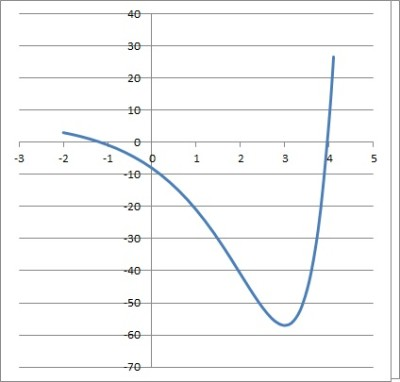The function 4x – 2x+3 + 7 = 0 is zero at x = 0 and x = log2(7).

#### Example 5: Solving A Nonlinear Equation

Let’s say we want to solve the following nonlinear equation:

• log6(x) + log6(2) = 3

This is a nonlinear equation that includes a logarithm (a logarithmic equation).  The first thing to notice is that we can use the rules of logarithms to combine the terms on the left side using logb(X) + logb(Y) = logb(XY):

• log6(2x) = 3

Now we rewrite the logarithmic equation in exponential form, using the fact that log(N) = E is equivalent to bE = N:

• 63 = 2x
• 216 = 2x
• 108 = x

So, the solution to this logarithmic equation is x = 108. We can verify this in the graph below: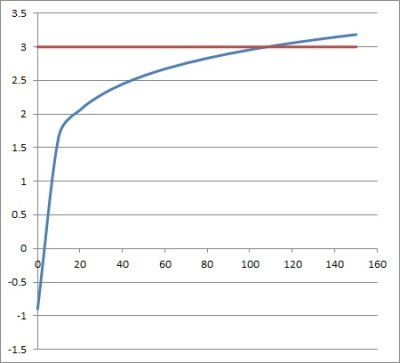The function y = log6(x)+ log6(2) (blue curve) intersects the function y = 3 (red line) at x = 108.

## What Is A Nonlinear System?

A nonlinear system contains two or more nonlinear equations.  For example, the following is a nonlinear system:

• y = x2
• y = 2x2 + 5x + 4

Both equations are nonlinear (quadratic equations).  Note that a nonlinear system can have three, four, or more equations (and three, four, or more variables).

### How To Solve A Nonlinear System

Let’s take a look at some examples of nonlinear systems, along with ways to solve them.

#### Example 1: Solving A Nonlinear System

Let’s say we want to solve the following nonlinear system:

• y = 3x2
• y = 5x2 + 6x + 4

We start by substitution y = 3x2 into the y in the second equation to get:

• 3x2 = 5x2 + 6x + 4

Then, we combine like terms to get:

• 0 = 2x2 + 6x + 4

We can divide by 2 to get:

• 0 = x2 + 3x + 2

Now, we can factor the right side to get:

• 0 = (x + 1)(x + 2)

So, the two solutions are x = -1 and x = -2. We can verify this in the graph below: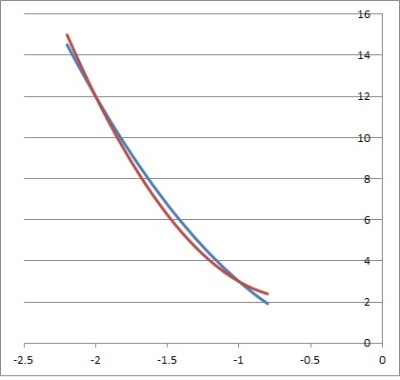The functions y = 3x2 (blue curve) and y = 5x2 + 6x + 4 (red curve) intersect at x = -1 and x = -2.

#### Example 2: Solving A Nonlinear System

Let’s say we want to solve the following nonlinear system:

• 4y = 2x
• log3(5y) = 6

We start by solving the first equation for y:

• 4y = 2x
• y = 2x/4

Then, we substitute y = 2x/4 into the y in the second equation to get:

• log3(5(2x/4)) = 6

Rearranging and simplifying, we get:

• log3((5/4)*(2x) = 6
• log3(1.25*2x) = 6

Using the definition of a logarithm, we get:

• 36 = 1.25*2x

Simplifying gives us:

• 729 = 1.25*2x
• 729/1.25 = 2x
• 583.2 = 2x

Taking the logarithm (base 2) on both sides give us:

• log2(583.2) = x

So, the solution is x = log2(583.2).

#### Example 3: Solving A Nonlinear System

Let’s say we want to solve the following nonlinear system:

• y = 3√x
• log4(y) = log2(3x)

We start by substituting the first equation into y in the second equation:

• log4(3√x) = log2(3x)

Now we rewrite 3√x as x1/3:

• log4(x1/3) = log2(3x)

Now we use a rules of logarithms:

• (1/3)*log4(x) = log2(3x)  [logb(XY) = Ylogb(X)]
• log4(x) = 3log2(3x)
• 4^(3log2(3x)) = x
• (22)^(3log2(3x)) = x
• 2^(6log2(3x)) = x
• (2^(log2(3x)))6 = x
• (3x)6 = x
• 729x6 = x
• 729x6 – x = 0
• x(729x5 – 1) = 0

One of the solutions is x = 0, and there are 5 possible solutions to 729x5 – 1 (at least one of them is real).

## Conclusion

Now you know what nonlinear equations and systems are.  You also know how to solve them, or how to find out when there are no solutions.